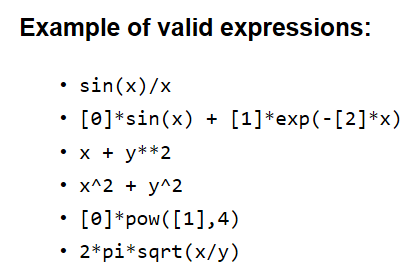# Accessing the value of pi in ROOT

Hi

I can’t access the value of pi in root though many tutorials/examples show its active use. I have also tried using TMath::pi

ROOT Version: Not Provided
Platform: Not Provided
Compiler: Not Provided

Hi,
in root you should use `TMath::Pi()`,

Cheers,
Stefano

I saw in many examples which used the constant simply by writing ‘pi’. Can you explain that in case?

In that screenshot of yours you do not seem to be in a ROOT session, it looks more like a `cmd.exe` session.

Can you maybe point us to such example?

yes I had accidentally uploaded the wrong pic, I have corrected it now

Can you point one of this example ? In such examples the variable `pi` should defined somewhere. Have a close look at the code.

The same point is discussed in documentation of Formula that how some names have their const values defined and can not be changed

Again can you point one of the examples where “pi” is used ? thanks.This is a snip from the same as mentioned
However I cannot yet find the use of pi in any of the examples.

This was apparently taken from
https://root.cern.ch/doc/master/classTFormula.html
, and it says the following below:

Formula may contain constants, eg:

• `sqrt2`
• `e`
• `pi`
• `ln10`
• `infinity`

, where by “Formula” the authors mean “a `TFormula` class object”. However, if you simply right `pi` in the ROOT prompt, this is not considered a `TFormula` class object.

yeah, apparently that would mean that TFormula:: pi would give us the value, right?

I should mention that one way of doing your work is define
auto pi = TMath::Pi(), but that is a different matter altogether.

No, the `pi` is not a member function of `TFormula`.

Then how does the page suggest we use it?
There are no clear examples

You can e.g. construct a `TF1` object using operations/functions (including the `pi`) defined in `TFormula`, for example:

``````root  TF1 *f1 = new TF1("f1", "x*pi" , 0, 5) // pi comes from TFormula
root  f1->Draw()
``````
``````root  auto f1 = new TF2("f2", "2*pi*sqrt(x/y)", 1, 5, 1, 5);
root  f2->Draw("surf2")
``````

This topic was automatically closed 14 days after the last reply. New replies are no longer allowed.